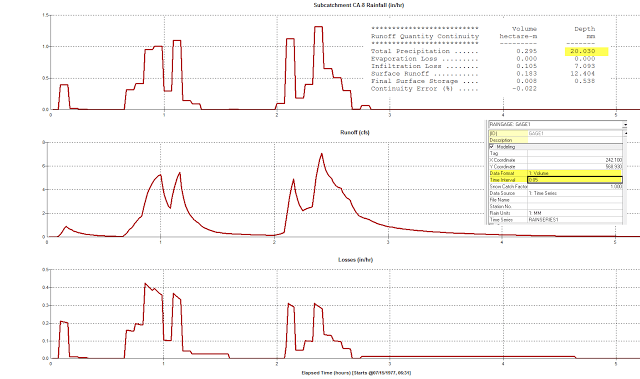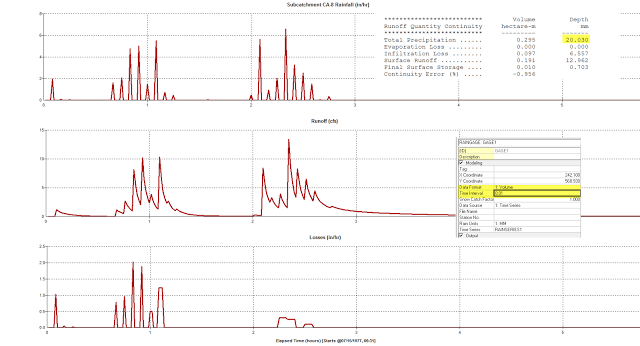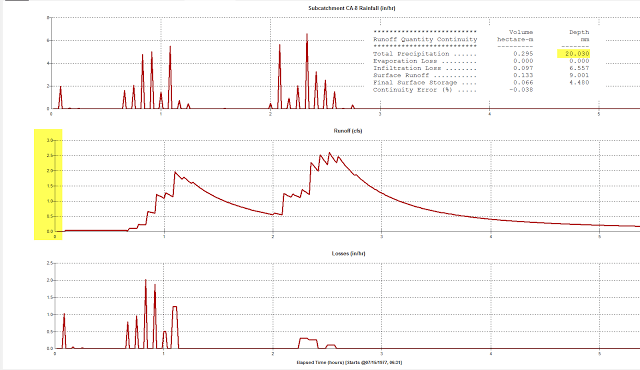#INFOSWMM

# The Effect of the Wrong Raingage Interval in H2OMap SWMM and InfoSWMM

Here is an example showing the Runoff, Rainfall and Infiltration losses when you use the wrong interval based on the hyetograph time series.

1.       Figure 1 shows the Rainfall, Runoff and Infiltration for a time interval of 5 minutes which matches the hyetograph interval, the raingage has units of mm and is the total volume over the interval

a.      The volume of rainfall is 20.03 mm as it the total rainfall over the interval
b.      The rainfall, runoff and infiltration graphs are smooth

2.      Figure 2 shows the Rainfall, Runoff and Infiltration for a time interval of 1 minutes which does NOT match the hyetograph interval, the raingage has units of mm and is the total volume over the interval

a.      The volume of rainfall is 20.03 mm as it the total rainfall over the interval
b.      The rainfall, runoff and infiltration graphs are not smooth as there is a gap of no rainfall between minute 1 and 5 for all of the hyetograph time intervals
c.       The peak rainfall is higher as the rainfall is over 1 minute instead of 5 minutes
d.      The peak runoff and infiltration rates are also higher but this difference depends on the roughness, depression storage and infiltration parameters of the catchment
e.      The slower the runoff the more Figure 1 and Figure 2 runoff will look alike even though the rainfall is the spiky versus smooth (Figure 3).Figure 1.  The Rainfall, Runoff and Infiltration loss for a gage interval of 5 minutes which matches the Time Series IntervalFigure 2.  The Rainfall, Runoff and Infiltration loss for a gage interval of 1 minutes which does not  match the Time Series IntervalFigure 3.  The Rainfall, Runoff and Infiltration loss for a gage interval of 1 minutes which does not  match the Time Series Interval, the Subcatchments Width is smaller than was used in Figure 1 and Figure 2

via Blogger http://www.swmm5.net/2013/10/the-effect-of-wrong-raingage-interval.html